# vICM DAYS AT MIT

June 13 - 14, 2022 12:00pm - 6:00pm MIT Building 2-190

The event will feature presentations of invited and plenary lectures of the upcoming virtual ICM.

## Program

### Monday, June 13th

• June 13th | 12:15-13:15

#### What is a Random Surface?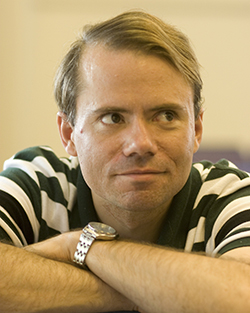Scott Sheffield (MIT)

Abstract

We will survey the modern theory of "random surfaces" while also reviewing the rich history of the subject and presenting numerous computer illustrations and animations. There are many ways to begin, but one is to consider a finite collection of unit equilateral triangles. There are finitely many ways to glue each edge to a partner, and we obtain a random sphere-homeomorphic surface by sampling uniformly from the gluings that produce a topological sphere. As the number of triangles tends to infinity, these random surfaces (appropriately scaled) converge in law. The limit is a "canonical" sphere-homeomorphic random surface, much the way Brownian motion is a canonical random path.

Depending on how the surface space and convergence topology are specified, the limit is the Brownian sphere, the peanosphere, the pure Liouville quantum gravity sphere, the bosonic string or a certain conformal field theory. All of these objects have concise definitions, and are all in some sense equivalent, but the equivalence is highly non-trivial, building on hundreds of math and physics papers over the past half century.

More generally, the "continuum random surface embedded in $d$-dimensional Euclidean space" makes a kind of sense for any $d \in (-\infty, 25]$. This story can also be extended to higher genus surfaces, surfaces with boundary, and surfaces with marked points or other decoration. These constructions have deep roots in both mathematics and physics, drawing from classical graph theory, complex analysis, probability and representation theory, as well as string theory, planar statistical physics, random matrix theory and a simple model for two-dimensional quantum gravity.

We present here a colloquium-level overview of the subject, which we hope will be accessible to both newcomers and experts. We aim to answer, as simply and cleanly as possible, the fundamental question. What is a random surface?

• June 13th | 13:15 - 14:15

#### Lunch

• June 13th | 14:15 - 15:15

#### Combinatorial Statistics and the Sciences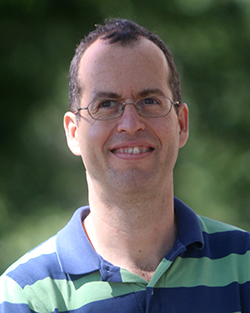Elchanan Mossel (MIT)

Abstract

Combinatorial Statistics studies inference in discrete stochastic models. Inference of such models plays an important role in the sciences. We survey research in Combinatorial Statistics involving broadcasting on trees. We review the mathematical questions that arise in the analysis of this process and its inference via "Belief Propagation". We discuss the mathematical connections to statistical physics, the social sciences, biological sciences and theoretical computer science.

• June 13th | 15:30 - 16:30

#### The Positive Grassmannian, the Amplituhedron, and Cluster Algebras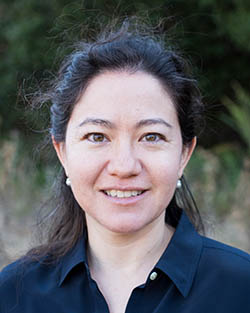Lauren Kiyomi Williams (Harvard)

Abstract

The positive Grassmannian Gr$^{\geq0}_{k,n}$ is the subset of the real Grassmannian where all Plücker coordinates are nonnegative. It has a beautiful combinatorial structure as well as connections to statistical physics, integrable systems, and scattering amplitudes. The amplituhedron $A_{n,k,m}(Z)$ is the image of the positive Grassmannian Gr$^{\geq0}_{k,n}$ under a positive linear map $\mathbb{R}^n \rightarrow \mathbb{R}^{k+m}$. We will explain how ideas from oriented matroids, tropical geometry, and cluster algebras shed light on the structure of the positive Grassmannian and the amplituhedron.

• June 13th | 16:45 - 17:45

#### Theory of Graph Neural Networks: Representation and Learning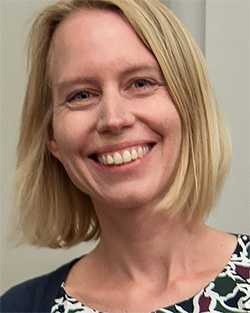Stefanie Jegelka (MIT)

Abstract

Graph Neural Networks (GNNs) are neural network architectures targeted at learning a map from graphs to a vector space. Due to their success in practice, they have become popular machine learning models for prediction tasks on nodes, graphs and configurations of points. In this talk, we summarize a selection of the emerging theoretical results on approximation and learning properties of widely used message passing GNNs and higher-order GNNs, focusing on function approximation, estimation and generalization, and extrapolation. Along the way, we touch upon various connections, including graph isomorphism, equivariant functions, combinatorial and local algorithms.

### Tuesday, June 14th

• June 14th | 12:15 - 13:15

#### Decoupling and Vinogradov's Mean Value Problem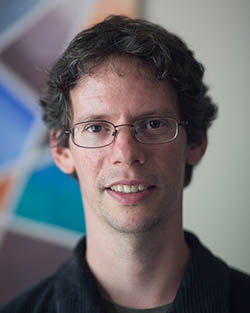Larry Guth (MIT)

Abstract

Decoupling is a recent development in Fourier analysis that has solved several longstanding problems. We will give an introduction to decoupling where we describe one of these problems and then describe some of the ideas of the proof.

Vinogradov's mean value problem is about the number of solutions of a certain system of diophantine equations, which can be rewritten as the moment of a certain trigonometric polynomial. It was raised in the 1930s and resolved in the last decade.

The main new idea in the proof of decoupling is to combine estimates from many different scales. We will try to describe this process and why it is helpful.

• June 14th | 13:15 - 14:15

#### Lunch

• June 14th | 14:15 - 15:15

#### Probability Theory for Random Groups Arising in Number Theory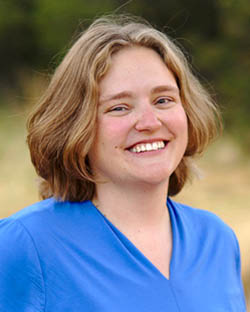Melanie Matchett Wood (Harvard)

Abstract

We consider the probability theory, and in particular the moment problem and universality theorems, for random groups of the sort of that arise or are conjectured to arise in number theory, and in related situations in topology and combinatorics. The distributions of random groups that are discussed include those conjectured in the Cohen-Lenstra-Martinet heuristics to be the distributions of class groups of random number fields, as well as distributions of non-abelian generalizations, and those conjectured to be the distributions of Selmer groups of random elliptic curves. For these sorts of distributions on finite and profinite groups, we survey what is known about the moment problem and universality, give a few new results including new applications, and suggest open problems.

• June 14th | 15:30 - 16:30

#### Fractal Uncertainty Principle and Quantum Chaos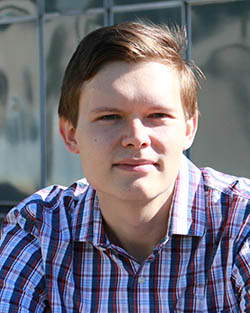Semyon Dyatlov (MIT)

Abstract

Classical/quantum correspondence tells us that for bounded times, singularities of solutions to wave/Schrödinger equations on a manifold propagate along geodesics. In this talk we go beyond this correspondence, showing results which rely on chaotic nature of classical dynamics but do not have classical counterparts, in particular:

• lower bounds on mass of high energy Laplacian eigenfunctions on negatively curved surfaces;
• observability for the Schrödinger equation on negatively curved surfaces;
• spectral gaps and exponential local energy decay of waves on convex co-compact hyperbolic surfaces.

A key new tool in the proofs is the fractal uncertainty principle, which states that no function can be localized close to a fractal set in both position and frequency. It is combined with a detailed understanding of classical/quantum correspondence up to the time when it fails.

This talk is based on joint works with Jean Bourgain, Long Jin, Stéphane Nonnenmacher, Joshua Zahl, and Maciej Zworski.